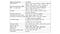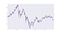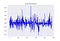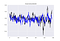# Neural networks for algorithmic trading. Hyperparameters optimization

Hello everyone! First of all I am thankful to you all, who is reading my blog, subscribing, and sharing opinions. It really makes me feel like what I do is not totally senseless and helps someone ❤.

In five last tutorials we were discussing financial forecasting with artificial neural networks where we compared different architectures for financial time series forecasting, realized how to do this forecasting adequately with correct data preprocessing and regularization, performed our forecasts based on multivariate time series and could produce really nice results for volatility forecasting and implemented custom loss functions. In the last one we have set and experiment with using data from different sources and solving two tasks with single neural network.

I think you have noticed that I usually take some architecture of the network as granted and don’t explain why I take this particular number of layers, this particular activation function, this loss function etc. This is really tricky question. Yes, it’s “normal” in deep learning community to take ReLU (or more modern in 2k17 alternative like ELU or SELU) as activation and be happy with this, but we usually don’t think if it’s correct. Talking about number of layers or learning rate for optimizer — we just take something standard. Today I want to talk about the way how to automatize the process of making a choice from these options.

## Previous posts:

As always, code is available on the Github.

# Hyperparameters searchTypical list of one single convolutional neural network training hyperparameters

Every machine learning has a lot of parameters to choose before starting to train a model, and in case of deep learning this list increases exponentially. You can see on the picture above typical list of parameters you pick from when you train some computer vision convolutional neural network.

But there is a way to automatize this! Very briefly, when you have a bunch of parameters and choices of their values, you can:

1. Launch Grid Search, trying to check EVERY possible combination of parameters and stop with one that optimizes your criteria (minimum of loss function for example)
2. Of course in most of cases you can’t wait so long, so Random Search is a good option, that looks on hyperparameter space randomly, but works faster and better mostly.
3. Bayesian optimization — we set a prior over hyperparameter distribution and sequentially update it while observing different experiments, which allows us to fit hyperparameters space better and, hence, find the minimum.

In this post we will consider last option as a black-box, concentrating on practical implementation and results analysis.

# HFT Bitcoin forecasting

I used data from Kaggle where user @Zielak posted last 5 years 1-minute prices data for Bitcoin.Sample plot from bitcoin prices

We will take a subset of last 10000 minutes and will try to build the best model to predict a change of price for next 10 minutes based on some historical period that we will choose later.

As input I want to take OHLCV tuple plus volatility and flatten this array in order to pass it to MLP model:

`o = openp[i:i+window]h = highp[i:i+window]l = lowp[i:i+window]c = closep[i:i+window]v = volumep[i:i+window]volat = volatility[i:i+window]x_i = np.column_stack((o, h, l, c, v, volat))x_i = x_i.flatten()y_i = (closep[i+window+FORECAST] - closep[i+window]) / closep[i+window]`

# Optimizing MLP parameters

For hyperparameter optimization we will use library Hyperopt, that gives easy interface for random search and Tree of Parzen Estimators (one variant of Bayesian optimization).

We simply need to define the space of hyperparameters (keys of dictionary) and sets of options for them (values). You can define a discrete choice of options (for activation functions options) or uniformly sample from some range (for learning rate):

`space = {'window': hp.choice('window',[30, 60, 120, 180]),        'units1': hp.choice('units1', [64, 512]),        'units2': hp.choice('units2', [64, 512]),        'units3': hp.choice('units3', [64, 512]),        'lr': hp.choice('lr',[0.01, 0.001, 0.0001]),        'activation': hp.choice('activation',['relu',                                                'sigmoid',                                                'tanh',                                                'linear']),        'loss': hp.choice('loss', [losses.logcosh,                                    losses.mse,                                    losses.mae,                                    losses.mape])}`

In our case I want to check if:

• more complex or less complex architecture do we need (number of neurons)
• activation function (let’s check in ReLU is really the best option)
• learning rate
• optimization criteria (maybe we can minimize logcosh or MAE instead of MSE)
• time window we need to pass into network to forecast next 10 minutes

And after we replace real parameters of layers, or data preparation, or training process with corresponding values of params dictionary. I suggest you to check whole code here.

`main_input = Input(shape=(len(X_train), ), name='main_input')x = Dense(params['units1'], activation=params['activation'])(main_input)x = Dense(params['units2'], activation=params['activation'])(x)x = Dense(params['units3'], activation=params['activation'])(x)output = Dense(1, activation = "linear", name = "out")(x)final_model = Model(inputs=[main_input], outputs=[output])opt = Adam(lr=params['lr'])final_model.compile(optimizer=opt,  loss=params['loss'])history = final_model.fit(X_train, Y_train,                   epochs = 5,                   batch_size = 256,                   verbose=0,                   validation_data=(X_test, Y_test),                  shuffle=True)pred = final_model.predict(X_test)predicted = predoriginal = Y_testmse = np.mean(np.square(predicted - original))sys.stdout.flush() return {'loss': -mse, 'status': STATUS_OK}`

We will check performance during first 5 epochs of training a network. After running this code, we will wait till 50 iterations (experiments) with different parameters will be executed and Hyperopt will choose the best option for us, which is:

`best: {'units1': 1, 'loss': 1, 'units3': 0, 'units2': 0, 'activation': 1, 'window': 0, 'lr': 0}`

It means that we want last two layers have 64 neurons and first — 512 neurons, use sigmoid as activation (wow, interesting), take typical learning rate (0.001) and take window just of 30 minutes to predict next 10. Hmmmm… Okay.

# Results

First I want to build a network with a “pyramid” pattern I usually take for new data. I also mostly start with ReLU as activation function and take standard for Adam optimizer learning rate 0.002:

`X_train, X_test, Y_train, Y_test = prepare_data(60)main_input = Input(shape=(len(X_train), ), name='main_input')x = Dense(512, activation='relu')(main_input)x = Dense(128, activation='relu')(x)x = Dense(64, activation='relu')(x)output = Dense(1, activation = "linear", name = "out")(x)final_model = Model(inputs=[main_input], outputs=[output])opt = Adam(lr=0.002)final_model.compile(optimizer=opt,  loss=losses.mse)`

Let’s check performance, blue is our forecast and black — original differences, MSE = 0.0005, MAE = 0.017:Result with my basic architecture

And now let’s check how model with parameters found by Hyperopt will perform on this data:

`X_train, X_test, Y_train, Y_test = prepare_data(30)main_input = Input(shape=(len(X_train), ), name='main_input')x = Dense(512, activation='sigmoid')(main_input)x = Dense(64, activation='sigmoid')(x)x = Dense(64, activation='sigmoid')(x)output = Dense(1, activation = "linear", name = "out")(x)final_model = Model(inputs=[main_input], outputs=[output])opt = Adam(lr=0.001)final_model.compile(optimizer=opt, loss=losses.mse)`Result with Hyperopt’s parameters

In this case, numerical results (MSE = 4.41154599032e-05, MAE = 0.00507) and visual look much better!

To be honest, I don’t think this is really good option, especially I don’t agree with such a short training time window, I still want to try with 60 minutes and I think that Log-Cosh Loss is much more interesting loss option for regression. But I will stick with sigmoid activation for now, because seems like this is the thing that boosted performance a lot.

`X_train, X_test, Y_train, Y_test = prepare_data(60)main_input = Input(shape=(len(X_train), ), name='main_input')x = Dense(512, activation='sigmoid')(main_input)x = Dense(64, activation='sigmoid')(x)x = Dense(64, activation='sigmoid')(x)output = Dense(1, activation = "linear", name = "out")(x)final_model = Model(inputs=[main_input], outputs=[output])opt = Adam(lr=0.001)final_model.compile(optimizer=opt,  loss=losses.logcosh)`

Here MSE is 4.38998280095e-05 and MAE = 0.00503, which is just slightly better than what we got with Hyperopt’s parameters, but visually it looks much worse (trends are totally missing).

# Conclusion

I strongly recommend you to use hyperparameters search for every model you train, whatever data you’re working with. Sometimes it leads to some unexpected results like a choice of activation function (what, really sigmoid in 2017?) or window range (I didn’t expect half an hour of historical information be better than one hour).

If you dive a bit deeper into Hyperopt options, you will see how you also can make search for number of hidden of layers, use or ignore of multitask learning and coefficients of loss functions. Basically, you just need to take subset of your data, think of parameters you want to tune and leave your computer for a while. This is a first step to automated machine learning :)

P.S.
Follow me also in Facebook for AI articles that are too short for Medium, Instagram for personal stuff and Linkedin!

Written by

## Alexandr Honchar

#### 🇺🇦 🇮🇹 AI entrepreneur and practitioner. Consulting, giving talks, teaching, writing. Contact me to collaborate alex.honchar@neurons-lab.com

Welcome to a place where words matter. On Medium, smart voices and original ideas take center stage - with no ads in sight. Watch
Follow all the topics you care about, and we’ll deliver the best stories for you to your homepage and inbox. Explore
Get unlimited access to the best stories on Medium — and support writers while you’re at it. Just \$5/month. Upgrade